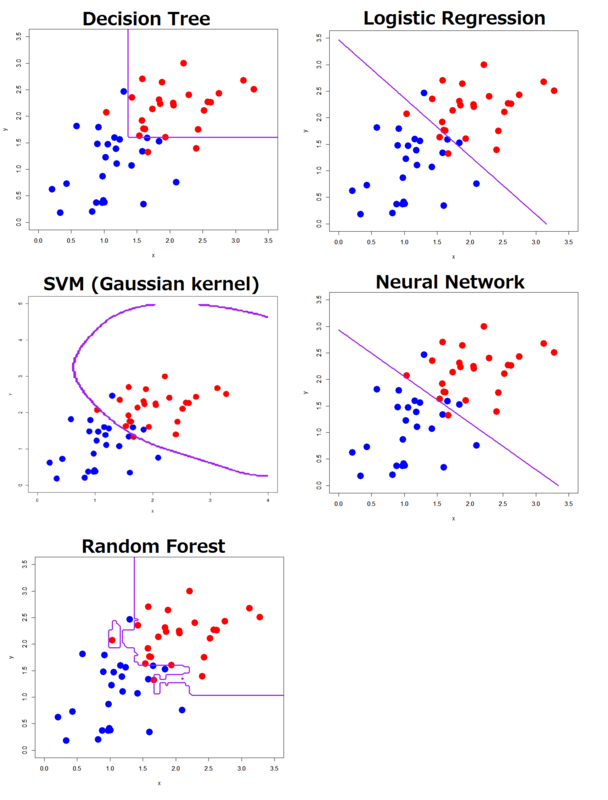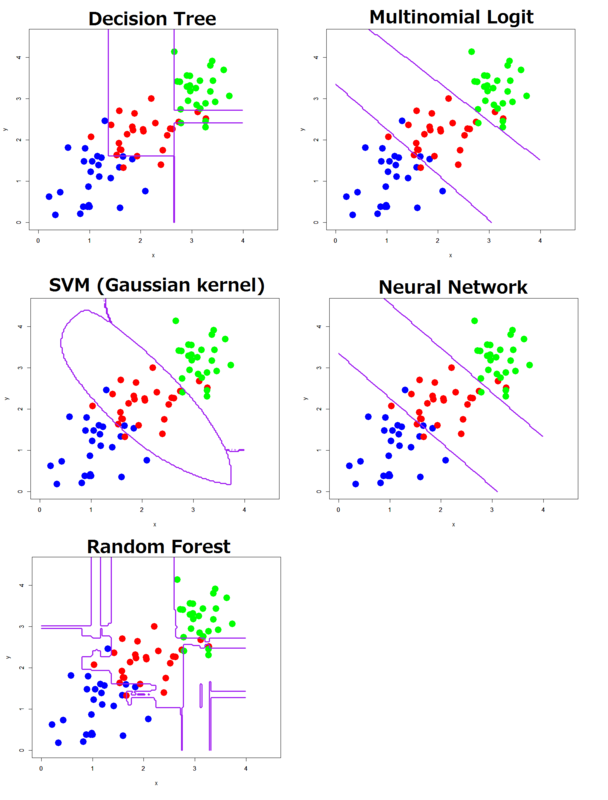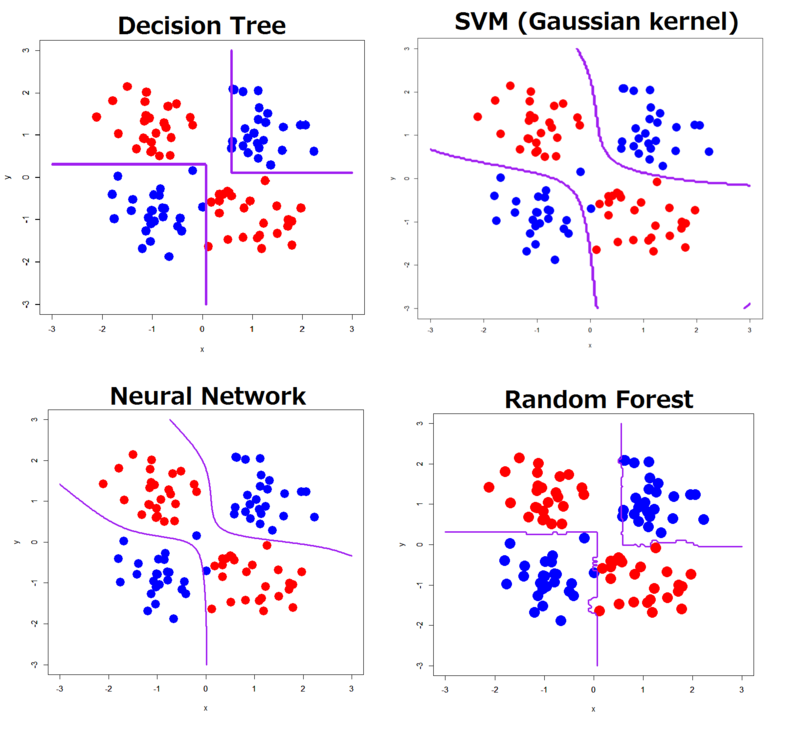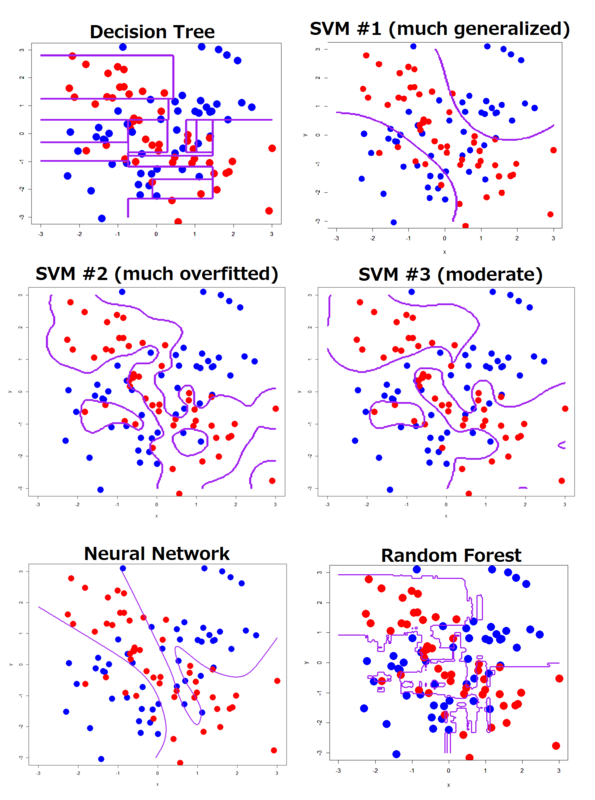# Comparing machine learning classifiers based on their hyperplanes or decision boundaries

In Japanese version of this blog, I've written a series of posts about how each kind of machine learning classifiers draws various classification hyperplanes or decision boundaries.

So in this post I want to show you a summary of the series and how their hyperplanes or decision boundaries vary (translated from Japanese version). It must be interesting and help you understand a nature of each classifier. Here I chose some representative classifiers as follows: decision tree (DT), logistic regression (LR: only for linearly separable cases), support vector machine (SVM), neural networks (NN: back-propagation multi-layer perceptron) and random forest (RF). They are all supervised learning methods and easy to import in R*1.

I'm still new to this field and just a "package-user", not serious expert in machine learning and its scientific basis*2. For such people, explaining meanings of algorithms or theorems is not helpful for understanding how they work -- instead, visualized feature (= hyperplanes or decision boundaries) well help us, I believe.

In order to overview how different their hyperplanes or decision boundaries are, I also arranged various 2D data sets: linearly separable (binary or 3-classes), linearly inseparable binary XOR (simple or complex) and linearly inseparable 4-classes XOR patterns. You can see easily it through these visualization of the hyperplanes or decision boundaries.

In each example below, I supposed that the true distribution were just a combination of 2, 3 or 4 two-dimensional normal distributions*3. Below are codes that generated the XOR patterns as an example.

```# XOR pattern (simple)
> p11<-cbind(rnorm(n=25,mean=1,sd=0.5),rnorm(n=25,mean=1,sd=0.5))
> p12<-cbind(rnorm(n=25,mean=-1,sd=0.5),rnorm(n=25,mean=1,sd=0.5))
> p13<-cbind(rnorm(n=25,mean=-1,sd=0.5),rnorm(n=25,mean=-1,sd=0.5))
> p14<-cbind(rnorm(n=25,mean=1,sd=0.5),rnorm(n=25,mean=-1,sd=0.5))
> t<-as.factor(c(rep(0,50),rep(1,50)))
> d1<-as.data.frame(cbind(rbind(p11,p13,p12,p14),t))
> names(d1)<-c("x","y","label")

# XOR pattern (complex)
> p21<-cbind(rnorm(n=25,mean=1,sd=1),rnorm(n=25,mean=1,sd=1))
> p22<-cbind(rnorm(n=25,mean=-1,sd=1),rnorm(n=25,mean=1,sd=1))
> p23<-cbind(rnorm(n=25,mean=-1,sd=1),rnorm(n=25,mean=-1,sd=1))
> p24<-cbind(rnorm(n=25,mean=1,sd=1),rnorm(n=25,mean=-1,sd=1))
> t<-as.factor(c(rep(0,50),rep(1,50)))
> d2<-as.data.frame(cbind(rbind(p21,p23,p22,p24),t))
> names(d2)<-c("x","y","label")
```

Two linearly separable patterns were merely a part of the XOR patterns above, except in the 3-classes case only I added one more two-dimensional normal distribution along y=x function.

We can evaluate how much each classifier got generalized and how precisely each one classified with looking at each decision boundary because already we know how the true distribution was*4; If each one follows well ture boundaries between quadrants*5, the one gets well generalized. At the same time you can easily see how many points get mis-classified. This is the purpose that I wrote this post. To simplify what we have to do, I omitted any cross-validation or classification tests for novel data; just looking at hyperplanes or decision boundaries is important for understanding how they work.

### Linearly separable pattern: binary (2-classes) classification

First, I'll show you a series of hyperplanes or decision boundaries by binary classification methods. This is the simplest and easiest classification problem -- but I feel their behaviors are much interesting.As expected, LR and NN worked well; in particular NN gave almost the same hyperplane as simple perceptron does. In linearly separable cases, NN can change itself to a variant of simple perceptron so that the hyperplane of NN looks very natural. There is no need to comment on LR: that is merely the best case for LR.

Interestingly, hyperplanes of DT, SVM (Gaussian kernel)((In this post I omitted SVM with linear kernel because the result is obvious :P))) and RF appear much worse. In particular for DT and RF, I think why they gave worse hyperplanes is that in principle their hyperplanes or decision boundaries must be drawn in parallel with x or y axis... they're far away from the correct decision boundary such that LR or NN gave. I'm afraid hyperplanes of DT, SVM and RF may be less generalized in this case.

### Linearly separable pattern: 3-classes classification

Almost all of classification functions in R can also handle multi-class problems*6. As a simple expansion, I ran 3-classes classifications with DT, LR, NN, SVM (Gaussian kernel), NN and RF.The result is more interesting. Hyperplanes or decision boundaries by DT, SVM and RF got further worse and more curious, even though still LR and NN gave clear 2 linear hyperplanes as expected. In multi-class cases, we may have to consider DT, SVM or RF can be almost never generalized... I feel, more classes, more problems of such classifiers in generalization "boosted".

### Linearly inseparable pattern: binary classification for a simple XOR pattern

I think XOR pattern should be the best for testing classifiers whether they can work in any linearly inseparable case. In particular, an advantage of NN over simple perceptron is that NN can correctly classify an XOR pattern although simple perceptron cannot. Here I omitted LR because LR doesn't work in linearly inseparable cases*7.The results were as I expected. DT, SVM and RF worked well. Only NN shows a little skewed hyperplanes... that slightly differ from the true boundaries. I have some concerns.

### LInearly inseparable pattern: binary classification for a complex XOR pattern

In addition to the XOR pattern above, this pattern includes some overlaps between quadrants. Such overlaps make it harder to classify than the simple pattern, of course.Some results look like joking. In particular those of SVM #2 and #3 were crazy, too overfitted, almost no generalized. On the other hand, SVM #1 was too generalized: yeah, it well followed the true boundaries, but its accuracy was bad (approx. 80%).

NN was not bad, but also a little overfitted. Accuracy of RF was great (100%) but looks a little overfitted too. I know it's hard to balance generalization and accuracy, but just in my opinion SVM #1 or #3 can be "not bad".

### 4-classes classification for a complex pattern

As already seen in the 3-classes case, most of classifiers in R packages provide multi-class classification methods. Of course, they can also solve more than 3-classes multi-classes problems.

Just for fun, I ran 4-classes classifications with DT, 3 versions of SVM, NN and RF. In order to evaluate their performance, I also wrote down classification accuracy of each classifier.

```> table(xor\$label,out2.xor4.rp.class)

0  1  2  3
0 17  0  2  6
1  0 20  3  2
2  0  1 23  1
3  0  1  1 23

# Decision trees: 83% accuracy

> table(xor\$label,predict(xor4.svm,xor[,-3]))

0  1  2  3
0 18  1  1  5
1  0 18  4  3
2  2  2 20  1
3  0  3  0 22

# SVM #1 (much generalized): 78% accuracy

> table(xor\$label,predict(xor4.svm2,xor[,-3]))

0  1  2  3
0 25  0  0  0
1  0 25  0  0
2  0  0 25  0
3  0  0  0 25

# SVM #2 (much overfitted): 100% accuracy

> table(xor\$label,predict(xor4.svm3,xor[,-3]))

0  1  2  3
0 25  0  0  0
1  0 24  1  0
2  0  0 25  0
3  0  0  0 25

# SVM #3 (middle): 99% accuracy

> table(xor\$label,out2.xor4.nnet.class)

0  1  2  3
0 20  1  1  3
1  0 19  3  3
2  1  2 21  1
3  1  4  0 20

# Neural Networks: 80% accuracy

> table(xor\$label,predict(xor4.rf,xor[,-3]))

0  1  2  3
0 25  0  0  0
1  0 25  0  0
2  0  0 25  0
3  0  0  0 25

# Random Forest: 100% accuracy
```The results well described how each classifier worked. As the 3-classes problem boosted wrong points of the classifiers in generalization, this 4-classes problem also seemed to boost them.

In terms of generalization, SVM #1, NN were better; they followed the true boundaries well, but their accuracy were bad. On the other hand, DT, SVM #2 & #3 were quite worse, too overfitted. Their hyperplanes or decision boundaries never reflected the true ones.

It was interesting that accuracy of RF was perfect (100%) but at the same time the global feature of the decision boundaries of RF seems to follow the true boundaries very well. Only RF showed a good balance between generalization and accuracy in this case.

### Summary

As well known in general, these results reminded me of importance of how to choose appropriate classifiers for each pattern. We have to apply LR or NN for linearly separable patterns, and SVM or RF for linearly inseparable patterns. In particular, choosing LR for linearly separable cases is very important; choosing worse classifiers for the case such as DT or RF can be harmful.

But in many cases, we don't know whether the dataset is linearly separable or inseparable. If so, choosing RF is better, I think. RF has a good balance of generalization and accuracy, and its computational cost is relatively low, even it can be distributed.

Personally, I want to learn more about NN; a data scientist colleague, who used to be a professional researcher on algorithms of NN, told me that NN is super flexible with tuning a lot of parameters. Once we enough understand how to tune them well, he says NN must be the most useful classifier and that's why NN is back as "Deep Learning".

### P.S.

A nice post by Justin (@) arrived as below. Thanks a lot!

The post gave almost the same comparisons using Python + scikit-learn except NN (but he added GMM classifiers). I know all of you will like it.

In addition, I found some media featured this post. For example:

http://www.datascienceassn.org/content/comparing-machine-learning-classifiers

*1:Functions and packages used here were: rpart(){mvpart} for decision tree, glm(){stats} or vglm(){MASS} for logistic regression or multinomial logit, svm(){e1071} for SVM, nnet(){nnet} for neural networks and randomForest(){randomForest} for random forest

*2:Even I'm Ph. D. in a certain experimental research field

*3:rnorm() function generated them

*4:Mixtures of 2D normal distributions arranged in some ways

*5:i.e. They are like a cross

*6:More than 2, even 4

*7:Indeed LR never works in linearly inseparable cases